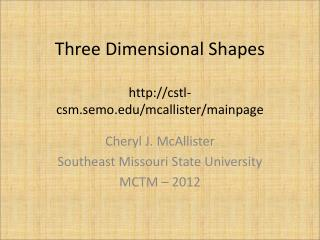Download PresentationThree Dimensional Shapes http://cstl-csm.semo.edu/mcallister/mainpage

# Three Dimensional Shapes http://cstl-csm.semo.edu/mcallister/mainpage - PowerPoint PPT PresentationDownload Presentation## Three Dimensional Shapes http://cstl-csm.semo.edu/mcallister/mainpage

- - - - - - - - - - - - - - - - - - - - - - - - - - - E N D - - - - - - - - - - - - - - - - - - - - - - - - - - -
##### Presentation Transcript

1. Three Dimensional Shapeshttp://cstl-csm.semo.edu/mcallister/mainpage Cheryl J. McAllister Southeast Missouri State University MCTM – 2012

2. While I am talking… • Select a color(s) of construction paper you like • Select a circle making tool of your choice • Draw circles with at least a 1 inch radius, but no more than a 2 in radius. • You will need at least 4 circles, but 8 will be the best. • You may have to take turns with the tools.

3. Geometry Standard for Grades Pre-K-2 http://www.nctm.org/standards/content.aspx?id=26846

4. Geometry Standard for Grades 3-5 http://www.nctm.org/standards/content.aspx?id=26814

5. Geometry Standard for Grades 6-8 • Instructional programs from prekindergarten through grade 12 should enable all students to— • Analyze characteristics and properties of two- and three-dimensional geometric shapes and develop mathematical arguments about geometric relationships  • Expectations: In grades 6–8 all students should— • precisely describe, classify, and understand relationships among types of two- and three-dimensional objects using their defining properties; • understand relationships among the angles, side lengths, perimeters, areas, and volumes of similar objects;

6. The activity today is one of many, many ways to get students thinking about and exploring 3-D figures • Can be used to review vocabulary • Can teach students to use construction tools such as compass and straight edge • Can be used to teach some math history • Can be used as an art activity to decorate the classroom

7. Polyhedron (polyhedra) • A three dimensional figure composed of polygonal regions (called faces) joined at the sides (edges). The point where edges meet is called a vertex.

8. Polyhedra are often categorized by their shapes • Prisms – composed of two bases (which are congruent polygons), joined by parallelograms (called lateral faces). • Pyramids – composed of 1 polygonal base and lateral faces that are triangles that meet at a single point called the apex.

9. Prisms

10. Other info about prisms • Prisms are often named for the shape of the bases IF the lateral faces are rectangles, then we have a right prism. IF the lateral faces are not rectangles, then we have an oblique prism. • The height (or altitude) of a prism is the perpendicular distance between the bases.

11. Triangluar Pyramid

12. More info about pyramids • Pyramids are often named by the shape of the base. • The height of a pyramid is the perpendicular distance from the apex to the base. • The slant height of a pyramid is the distance from the apex along a lateral face of the pyramid, perpendicular to the opposite edge of the face. (see next slide)

13. Height and slant height h = height s = slant height

14. More facts about pyramids • IF the apex is over the center of the base, then the pyramid is a right pyramid, if not, then the pyramid is oblique. • IF the base of the pyramid is a regular polygon, then the pyramid is said to be a regular pyramid.

15. Height of an oblique pyramid Note: the height is outside the pyramid

16. Regular polyhedra (Platonic solids) Tetrahedron Hexahedron Octahedron Dodecahedron Icosahedron

17. Extensions of this lesson • There are only 5 possible regular polyhedra. Can you explain why? • Investigate Euler’s Formula F + V = E + 2

18. Where to find directions • http://www.auntannie.com/Geometric/PlatonicSolids/

19. Another method - Folding nets • This is what a tetrahedron looks like if you flatten it out.

20. Thank you! cjmcallister@semo.edu http://cstl-csm.semo.edu/mcallister/mainpage